## Point Neurons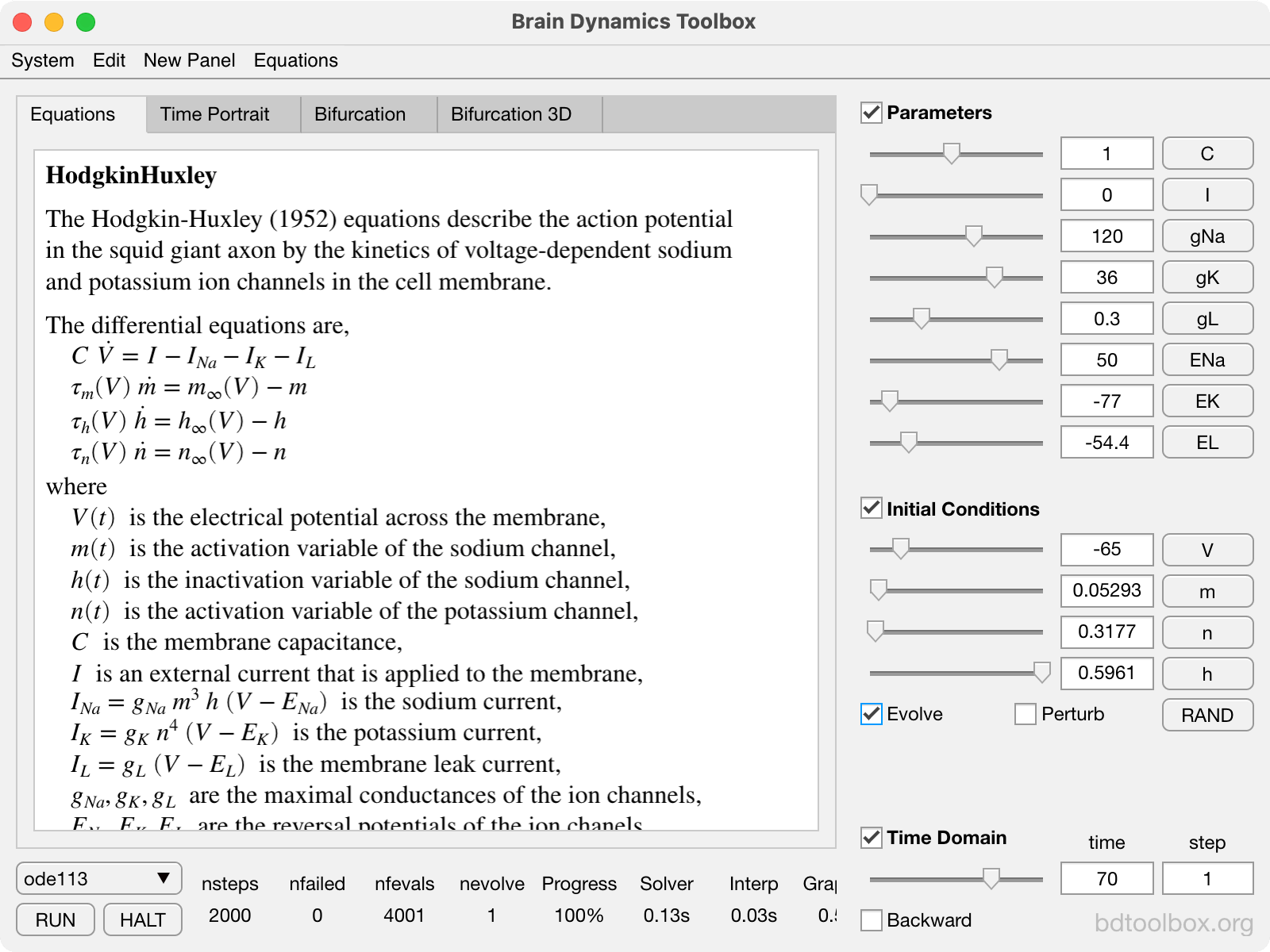Bistability in the Hodgkin-Huxley model of the action potential in squid axon.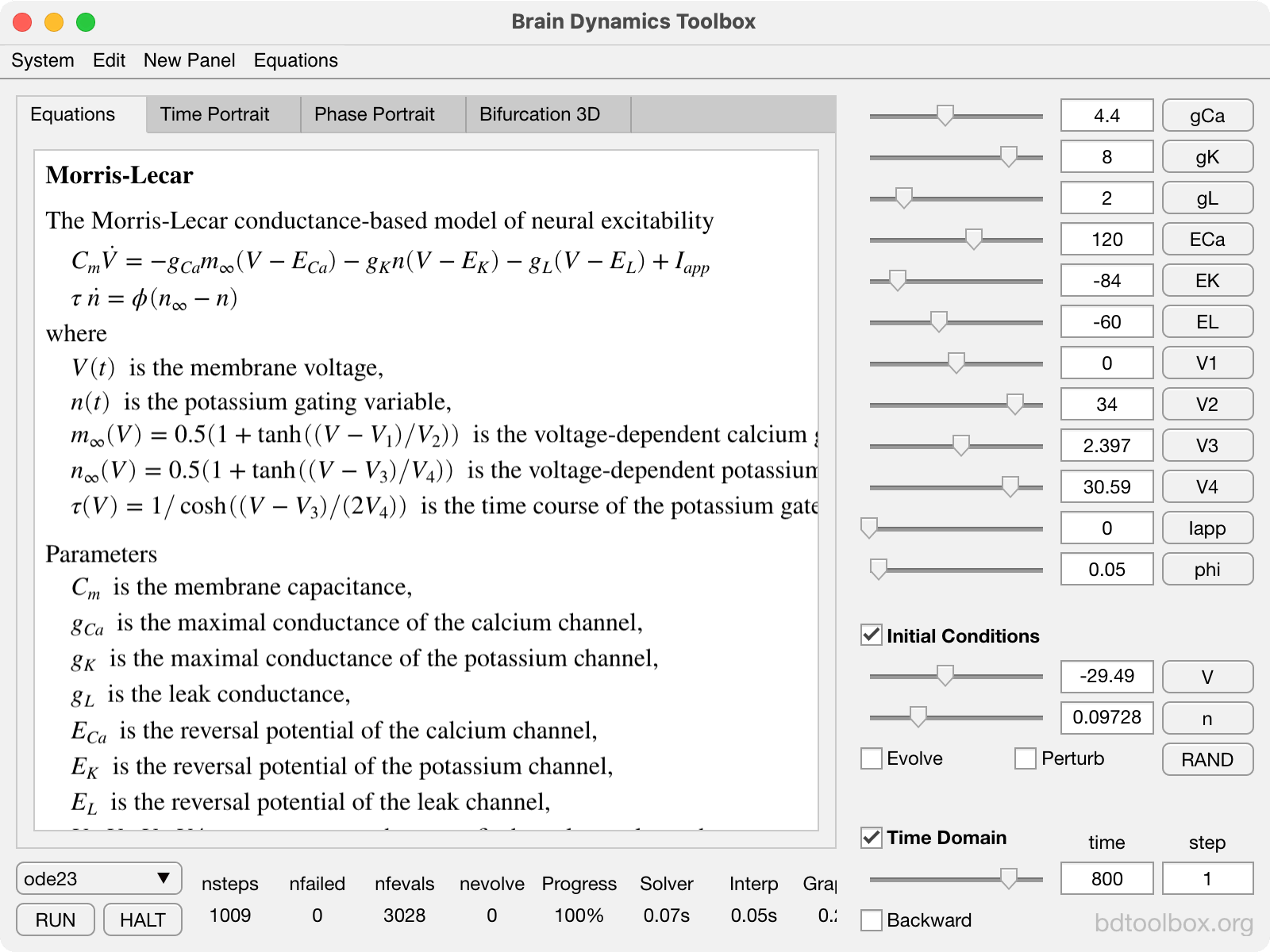Supercritical Hopf bifurction in the Morris-Lecar model of barnacle muscle.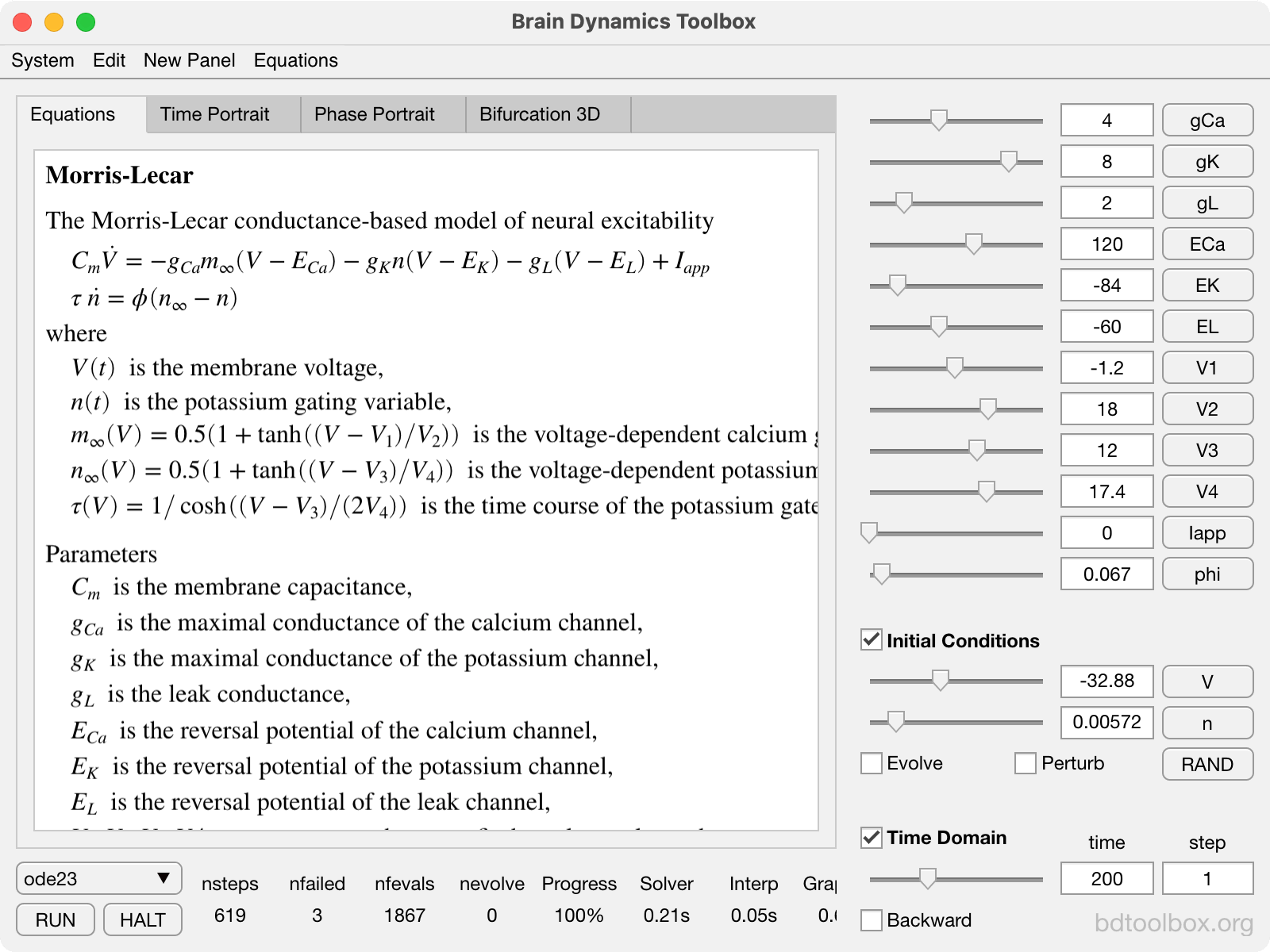Saddle-Node bifurcation on a Limit Cycle in the Morris-Lecar model.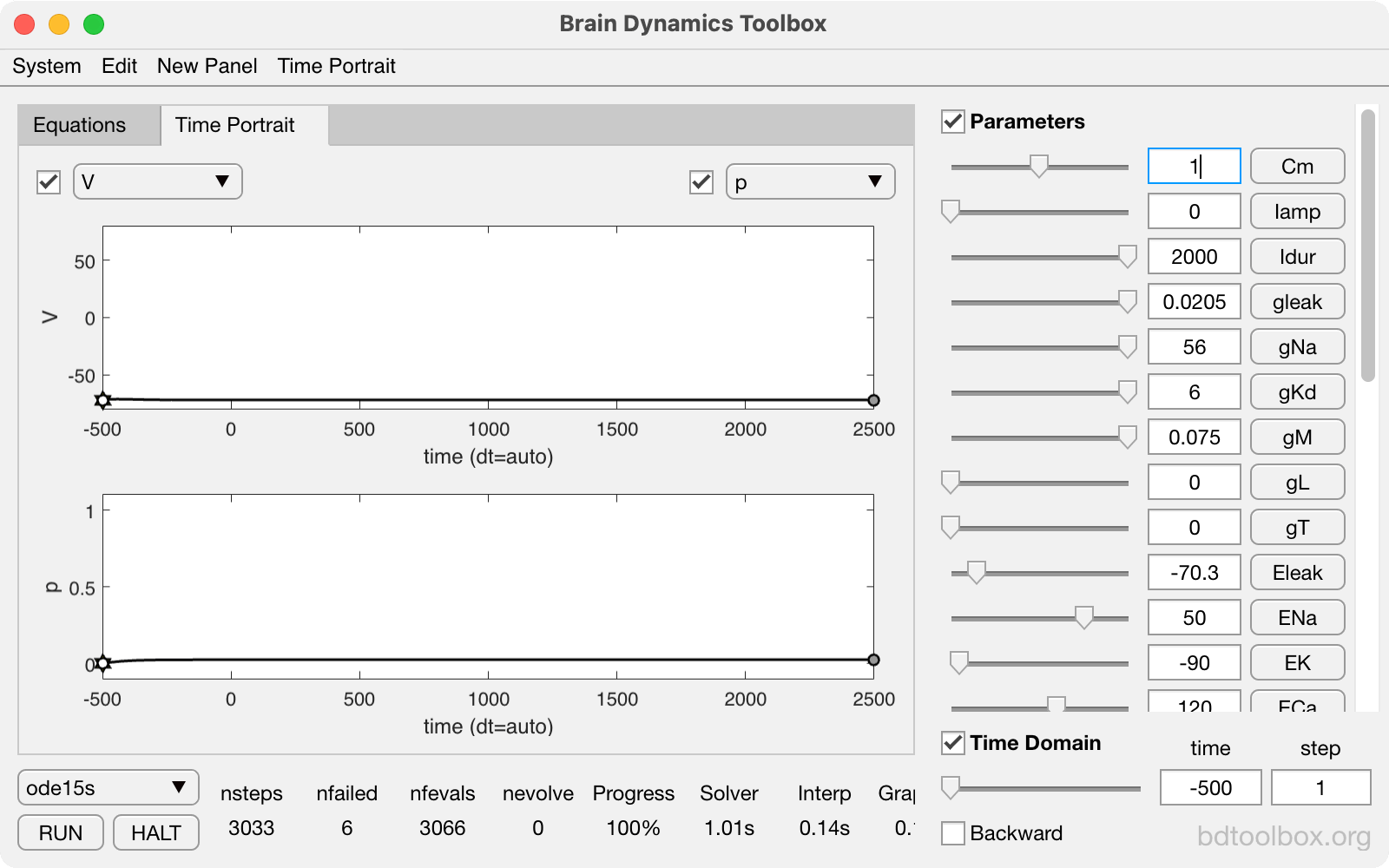Regular spiking in a minimal neural model by Pospischil, et al.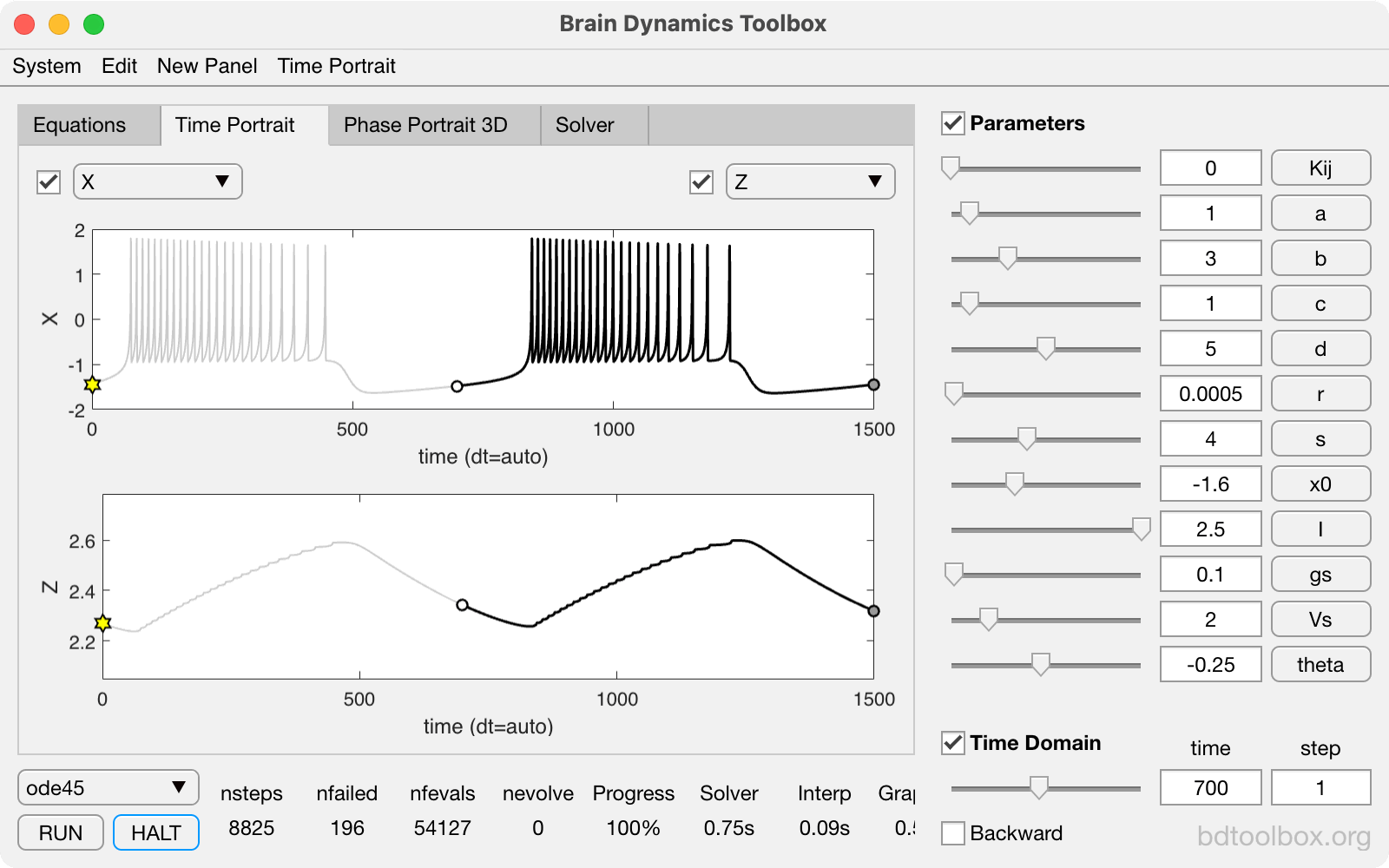Intrinsic Bursting in the Hindmarsh-Rose neuron.

## Neural Masses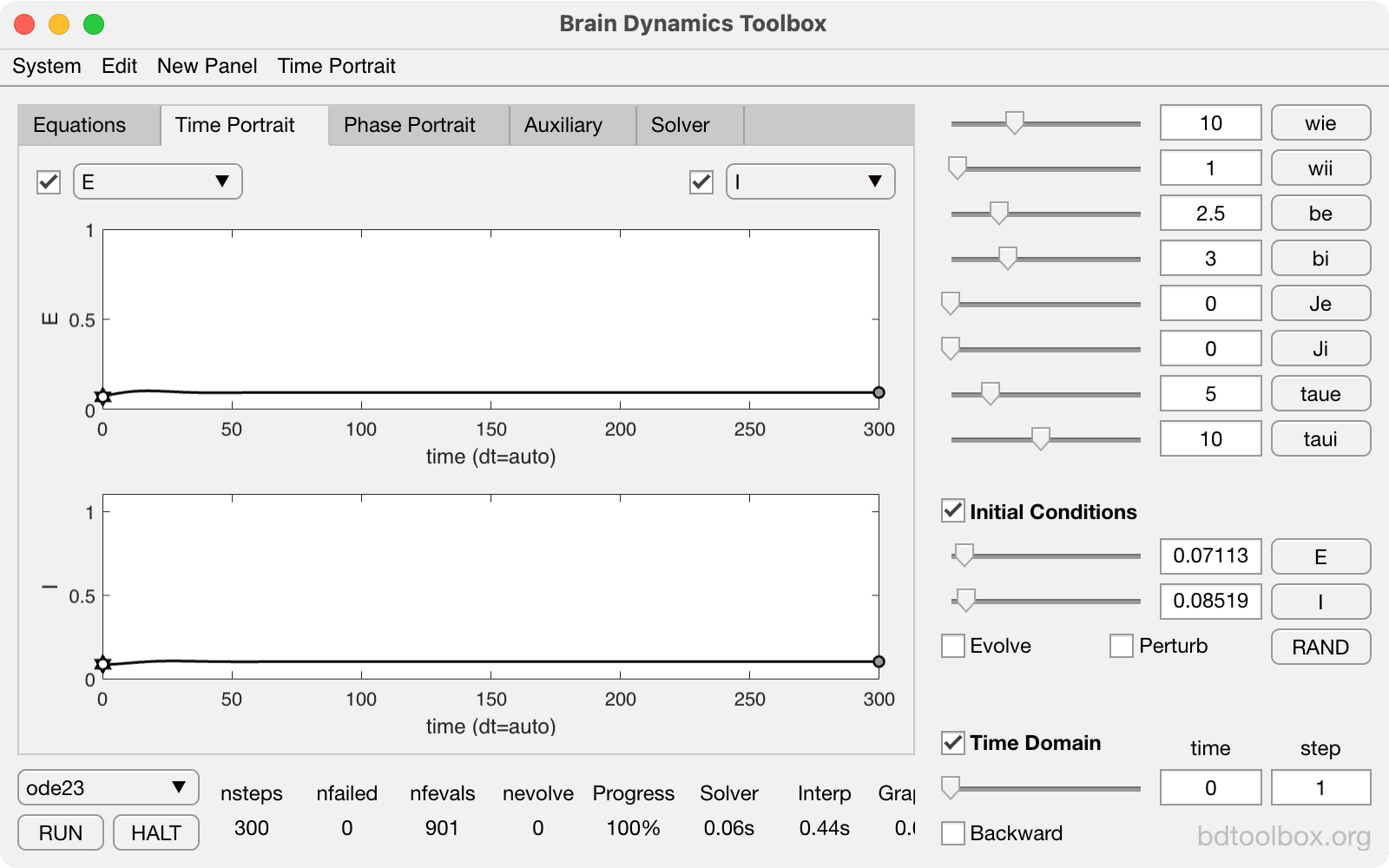Wilson-Cowan model of reciprocally-coupled excitatory and inhibitory neural populations.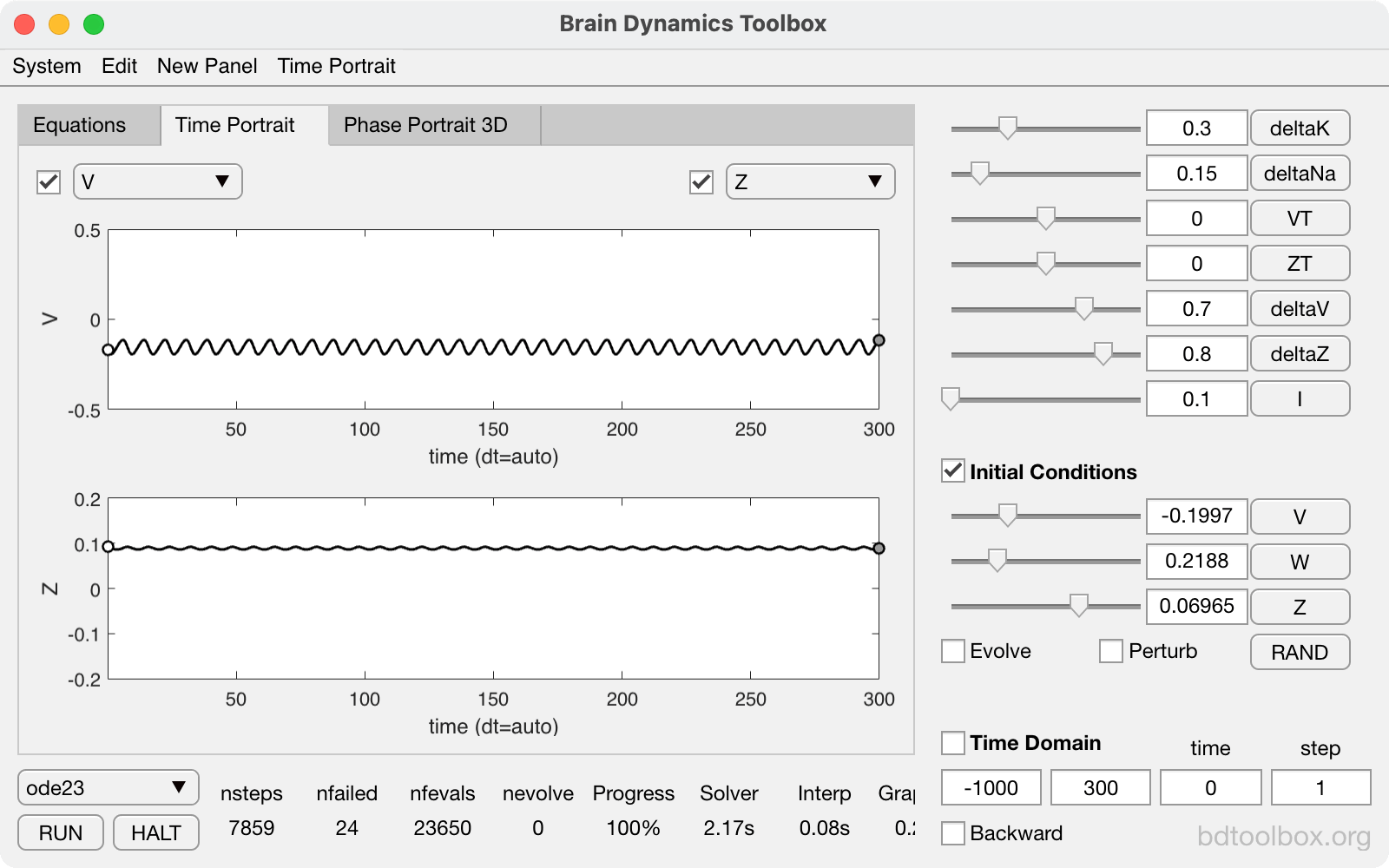Chaos in the Breakspear-Terry-Friston model.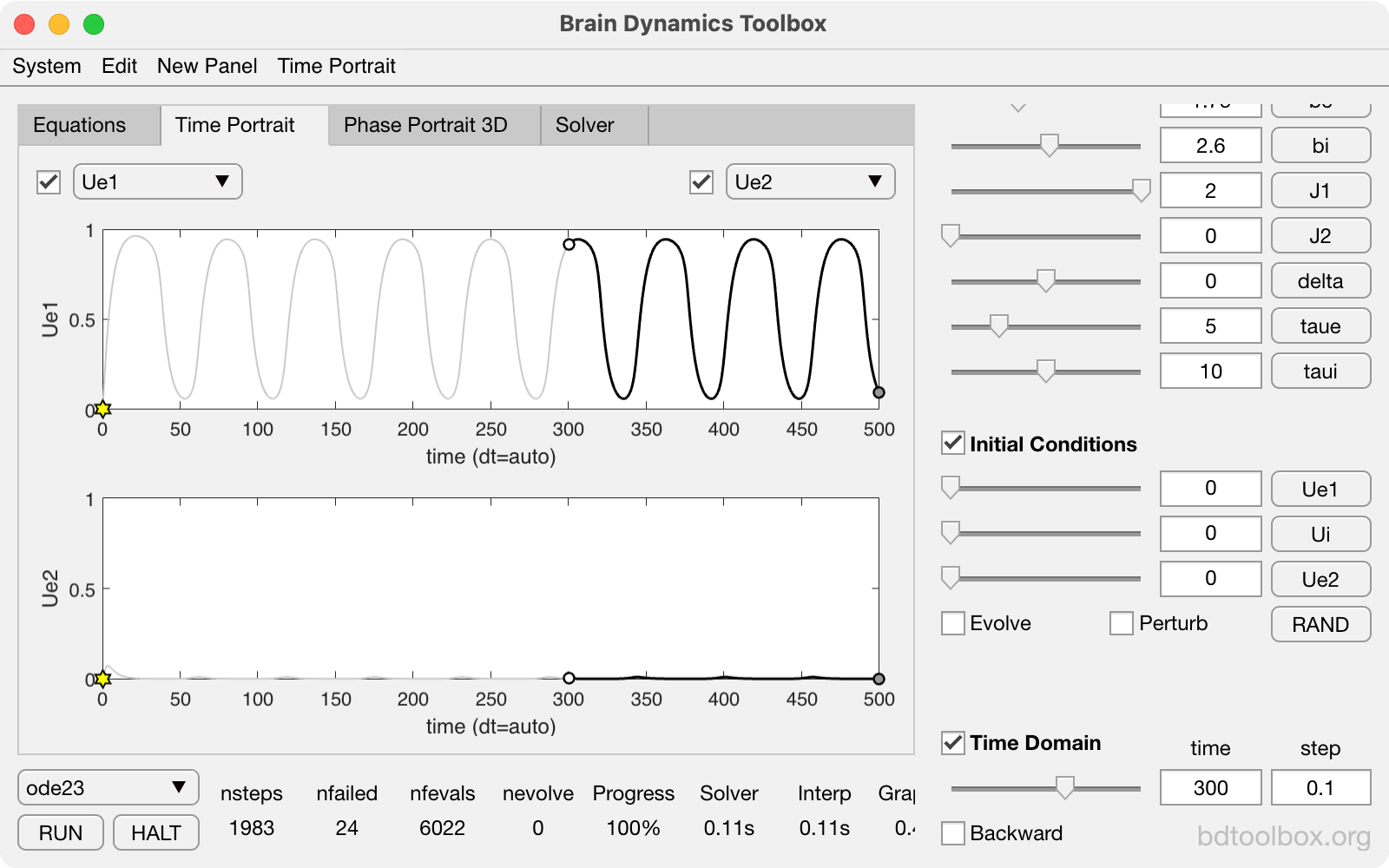Perceptual opponency in the Heitmann-Ermentrout E-I-E model.

## Spatial Models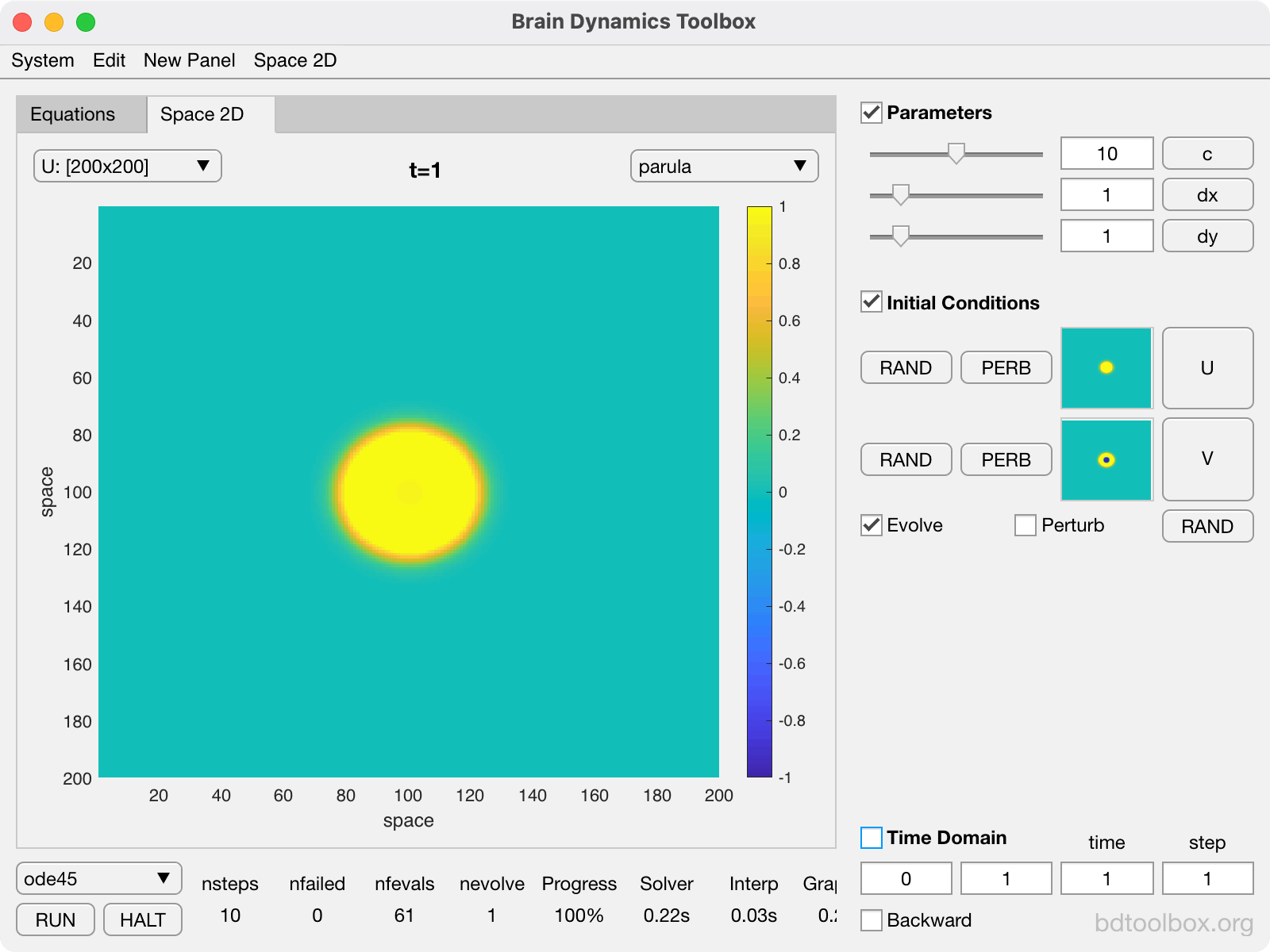The 2D Wave Equation with reflecting boundary conditions.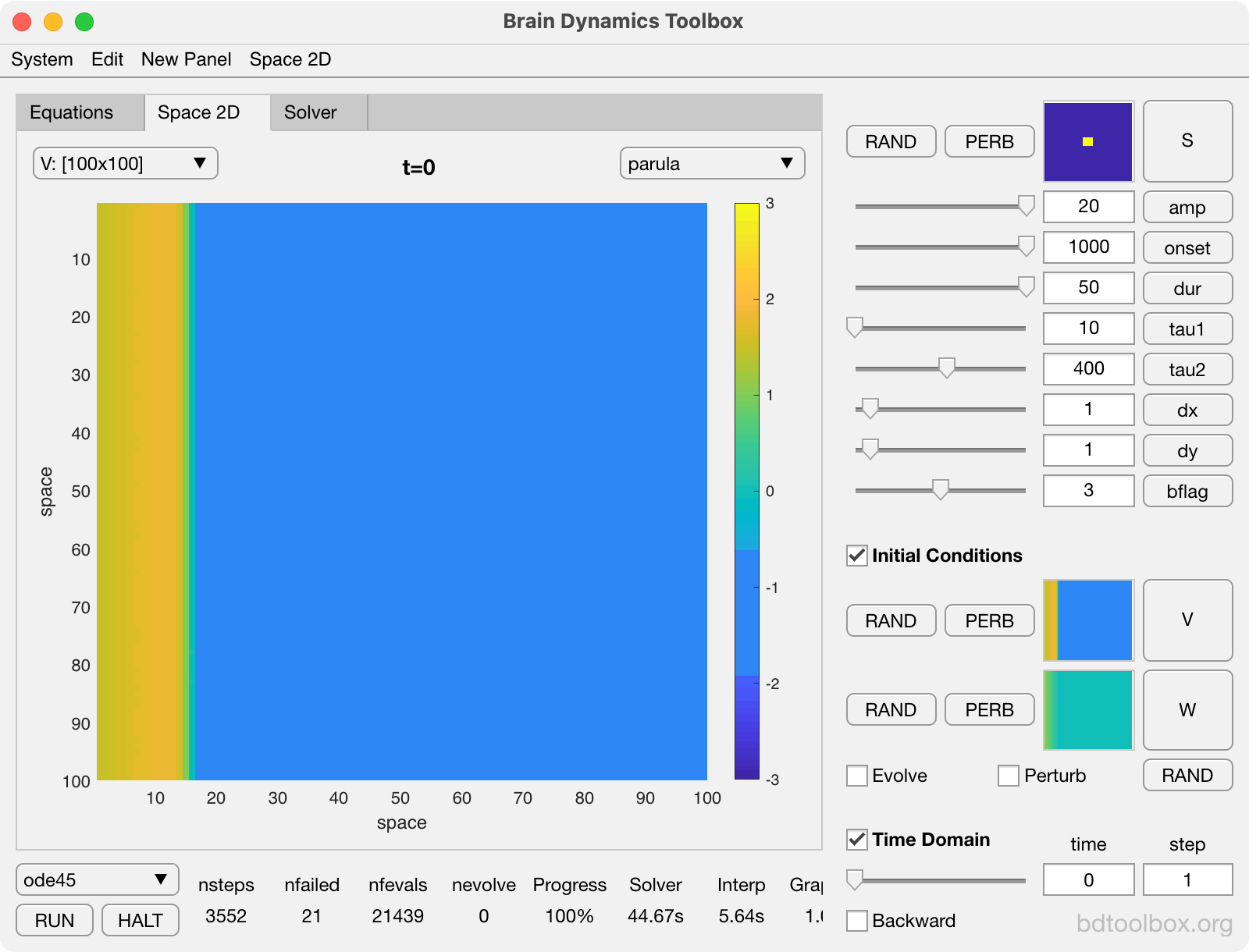Initiation of spiral rotors in a sheet of FitzHugh-Nagumo neurons.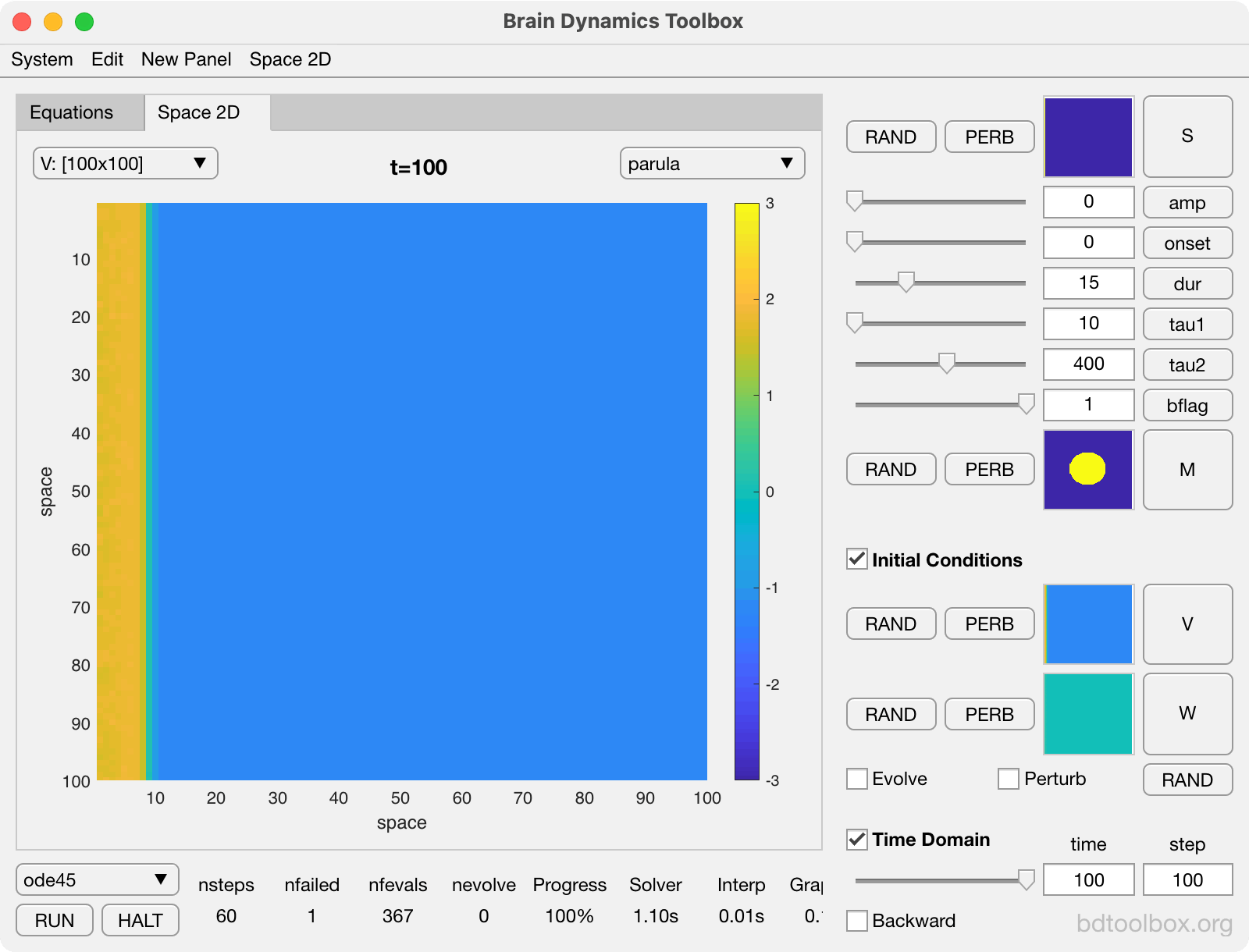Arrhythmogenesis in a heterogeneous sheet of FitzHugh-Nagumo neurons with a tissue boundary.

## Normal Forms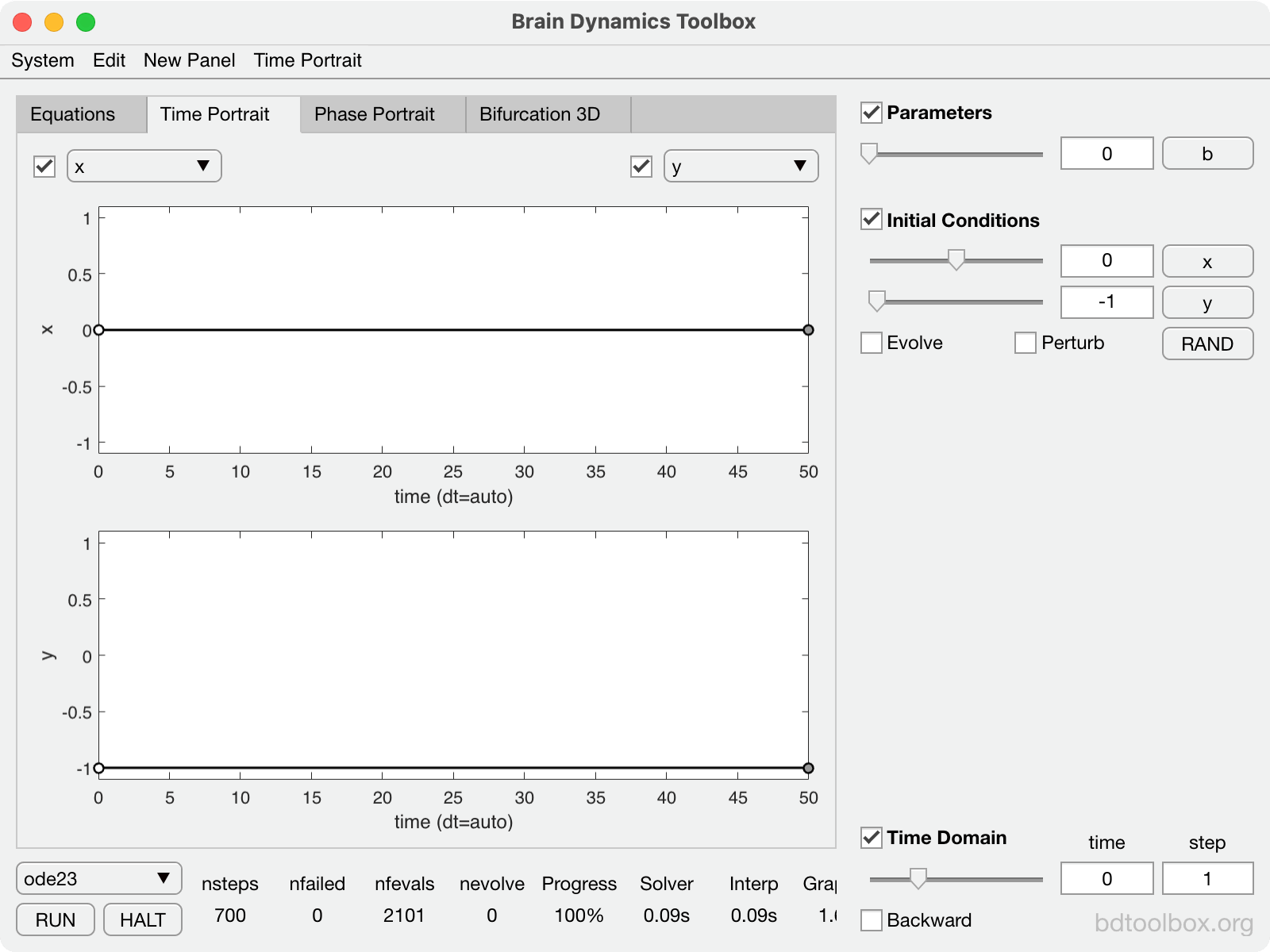Normal form of the Saddle-Node bifurcation on a Limit Cycle. The basis of Type-1 neural excitability.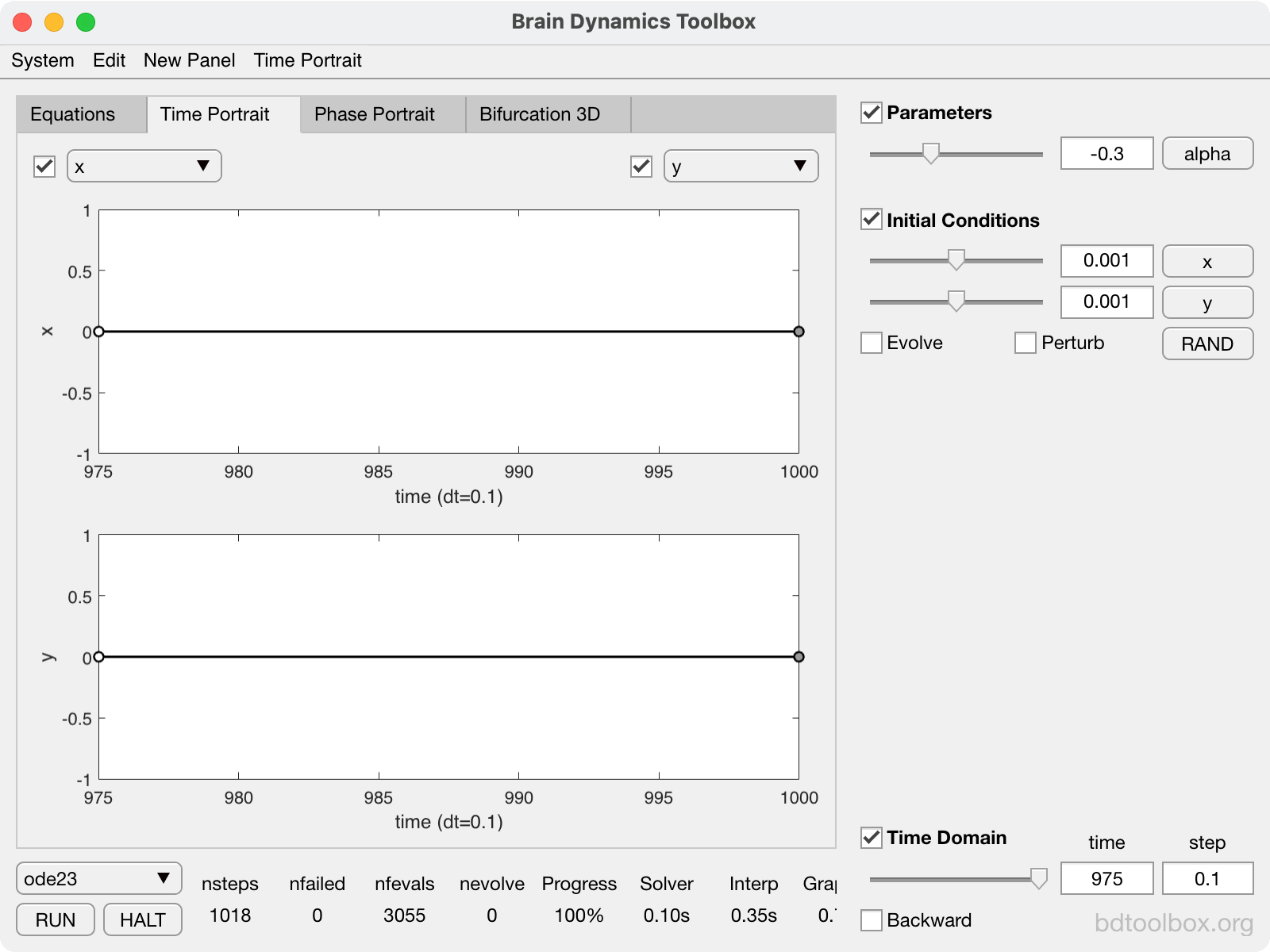Normal form of the Supercritical Hopf bifurcation. The basis of Type-2 neural excitability.

## Classics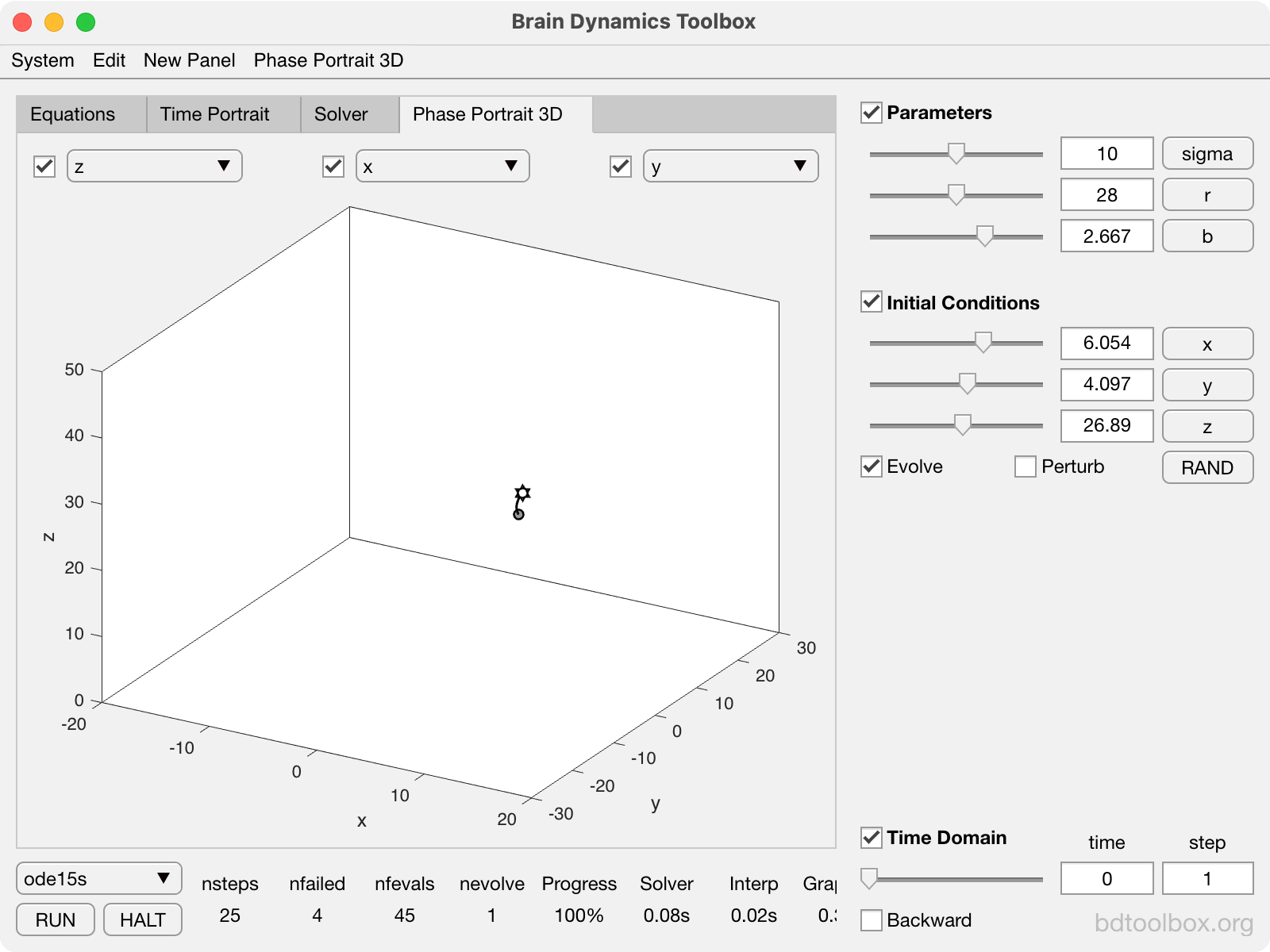Determistic chaos in the Lorenz Attractor.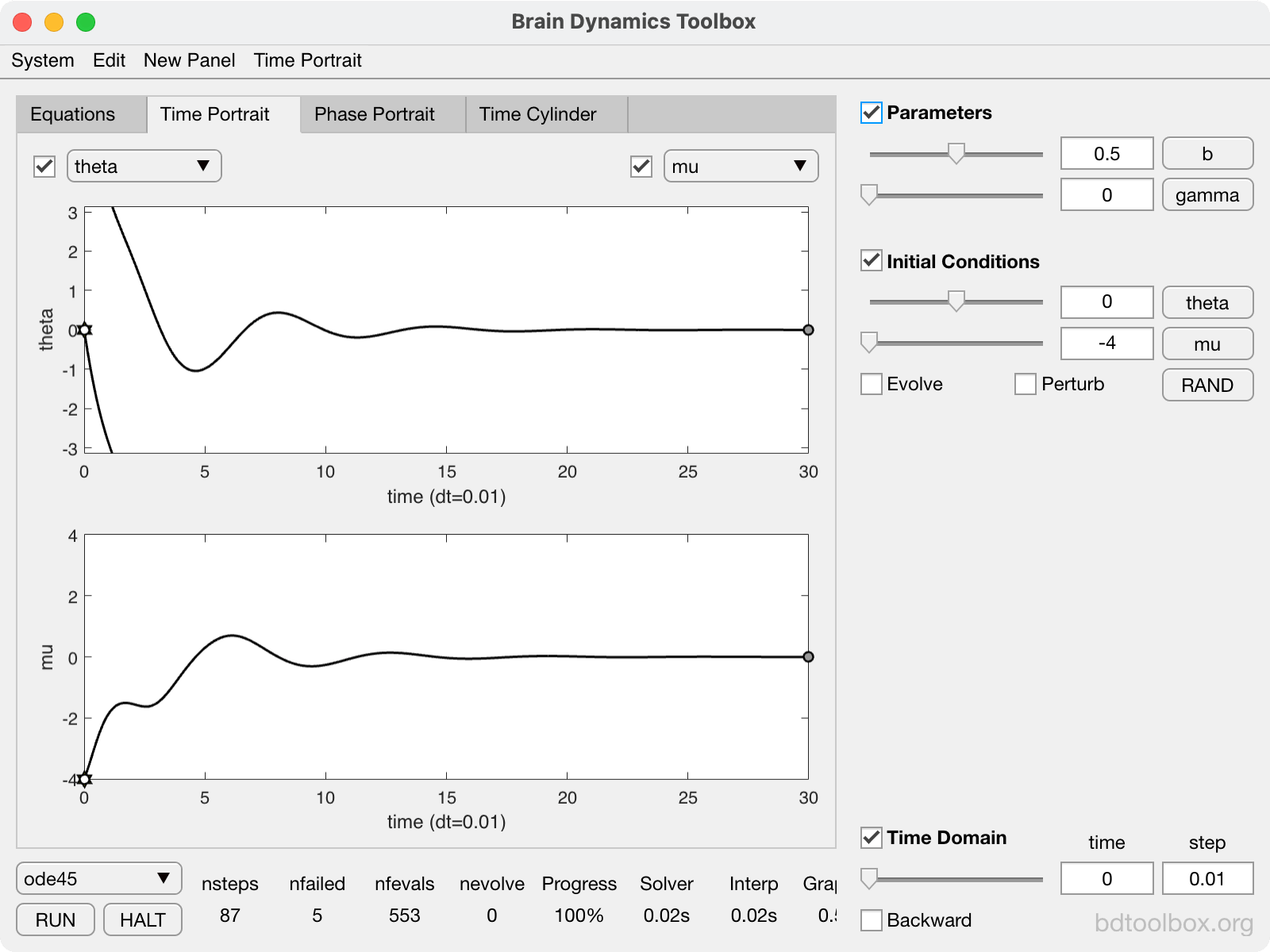The damped-and-driven pendulum.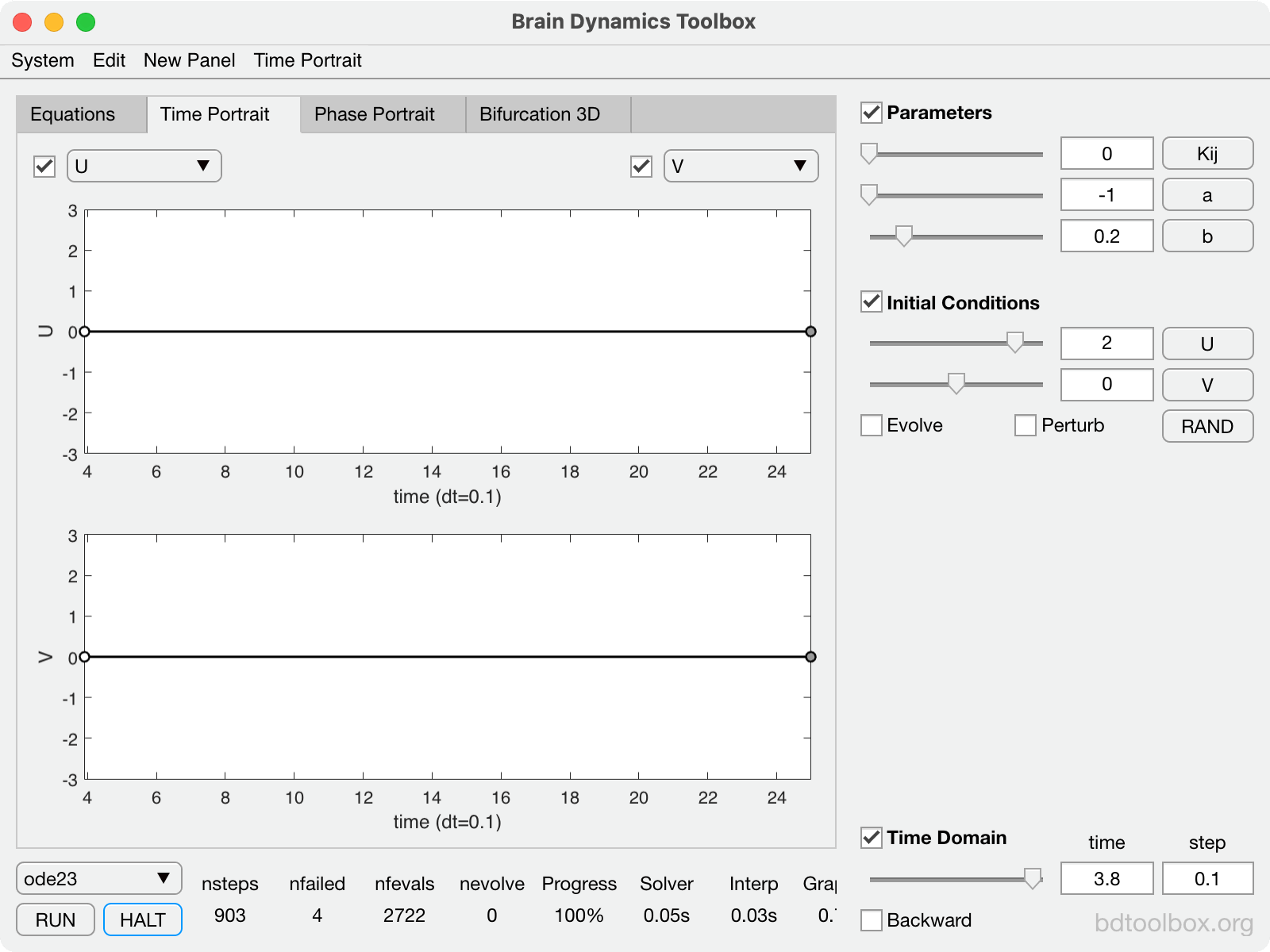The Van Der Pol relaxation oscillator.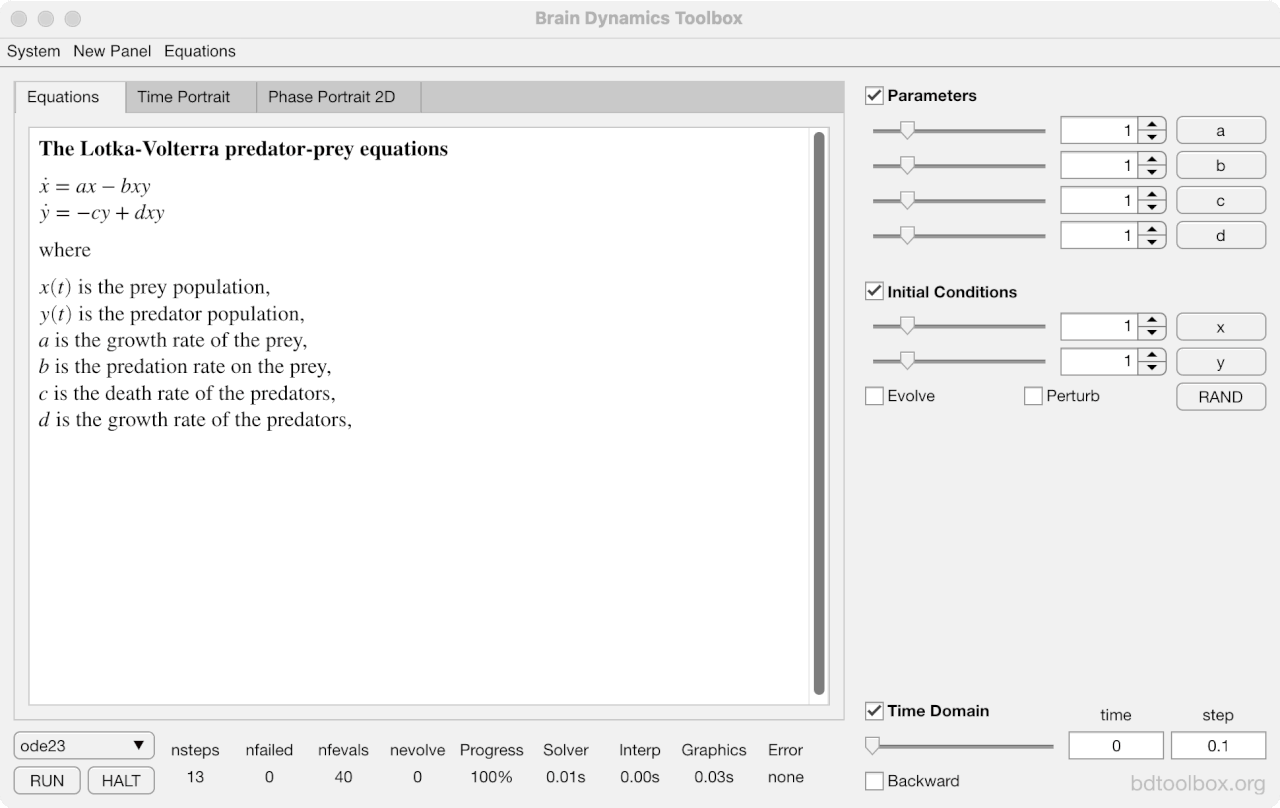The Lotka-Volterra predator-prey equations.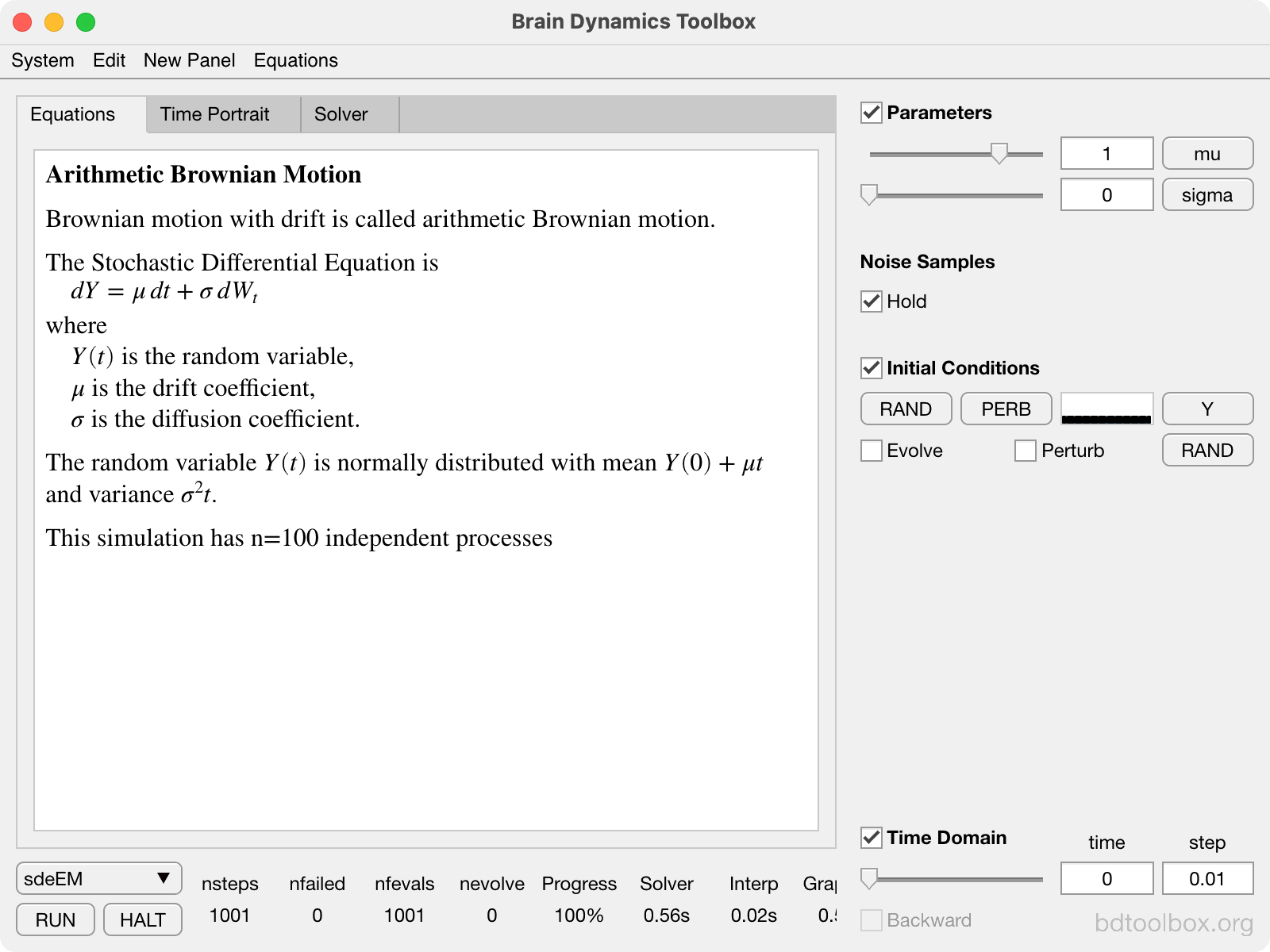Arithmetic Brownian Motion. Drift and diffusion.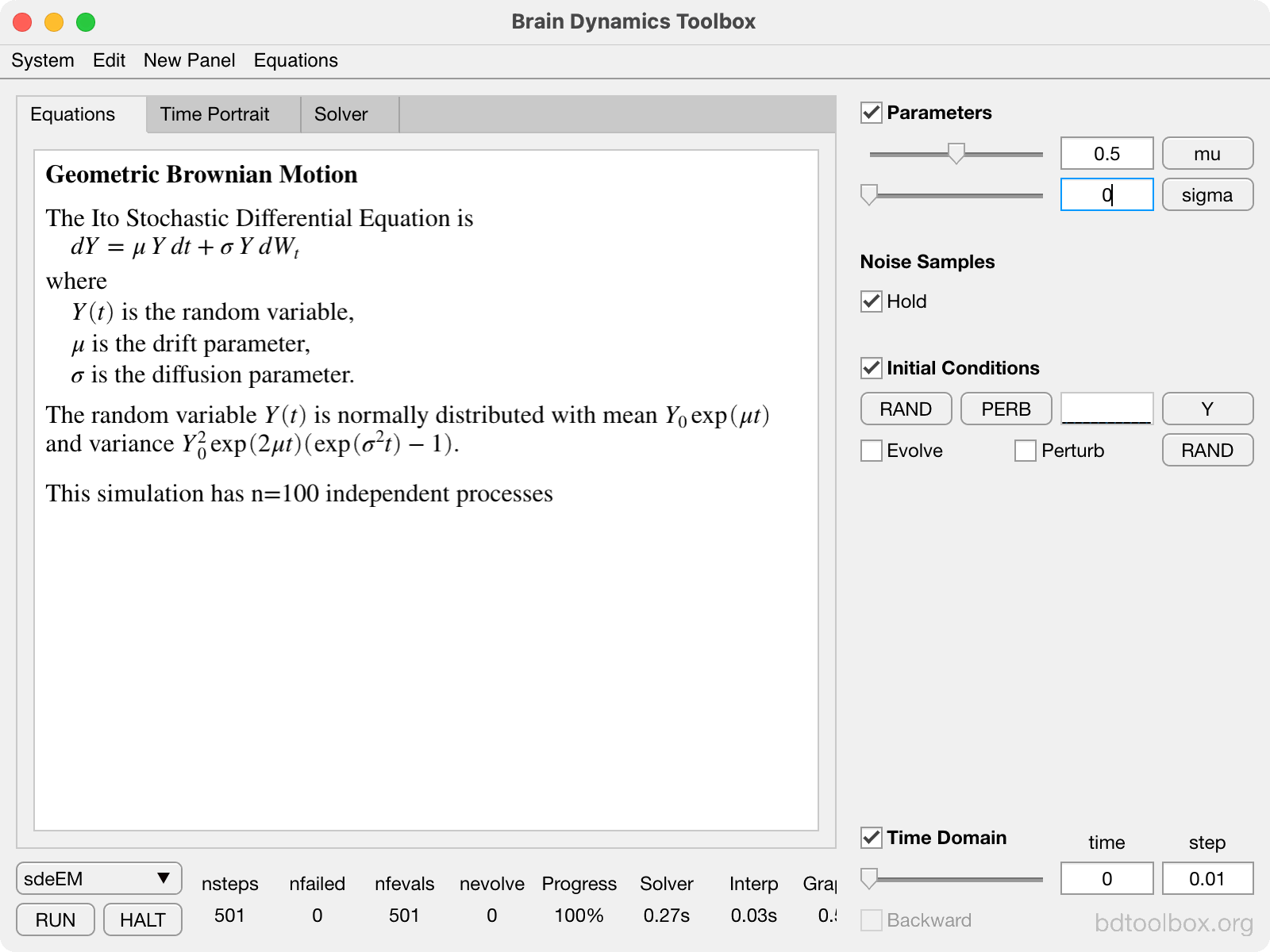Geometric Brownian Motion. Multiplicative noise. Mathematical finance.

## Strogatz Textbook Examples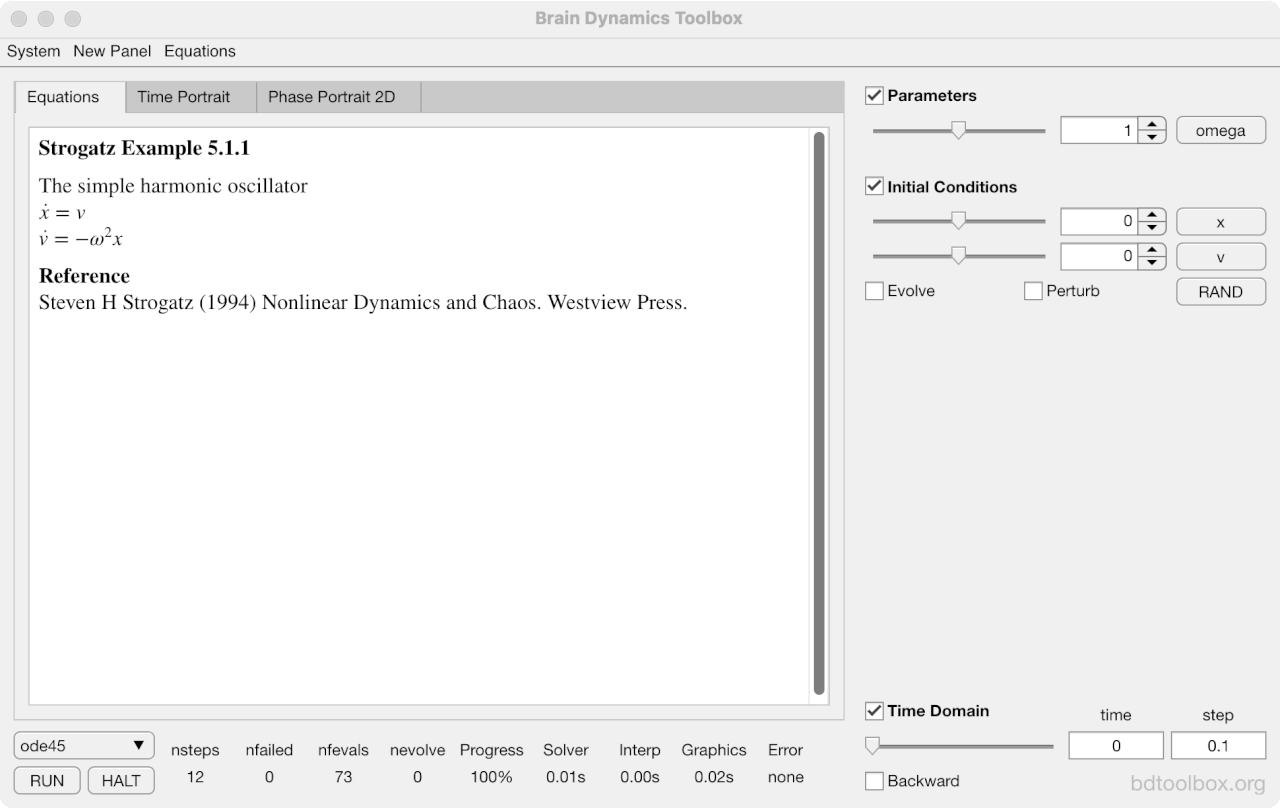Example 5.1.1. Closed orbits in the simple harmonic oscillator.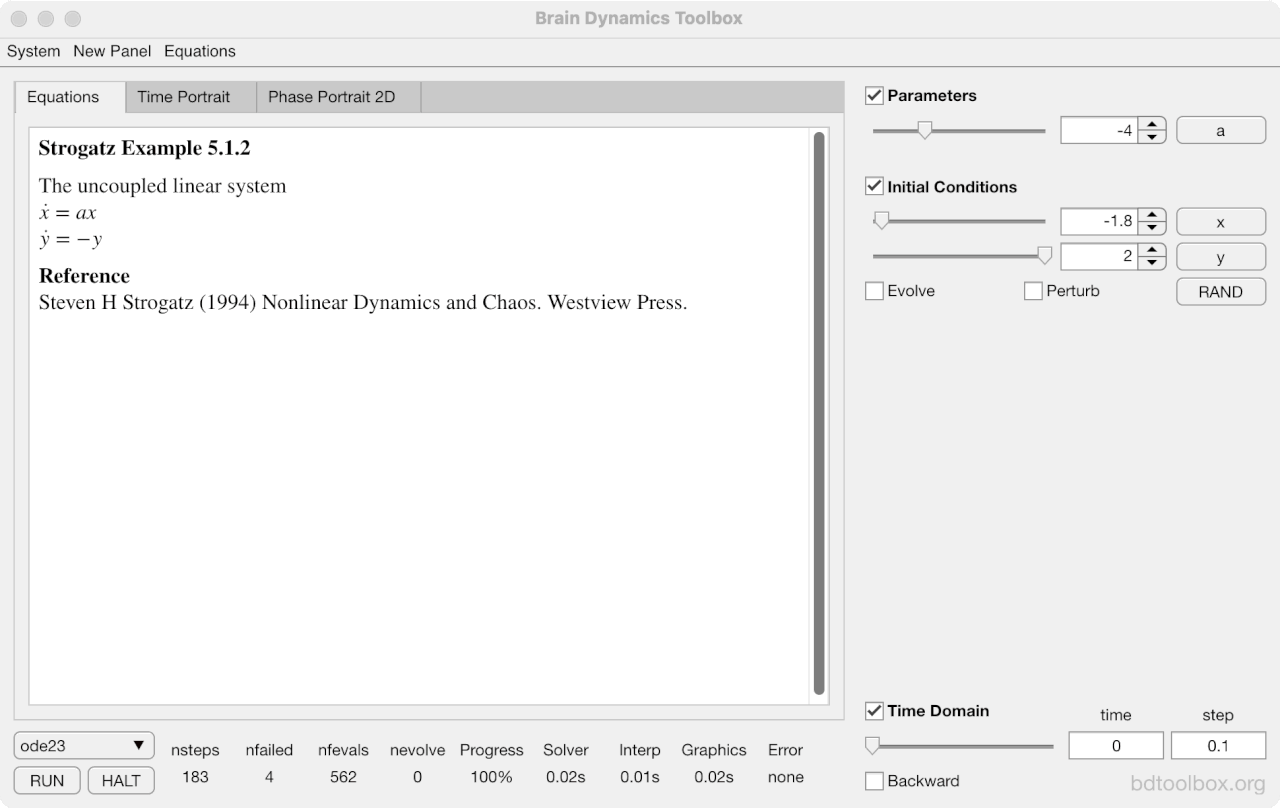Example 5.1.2. A pair of uncoupled linear equations.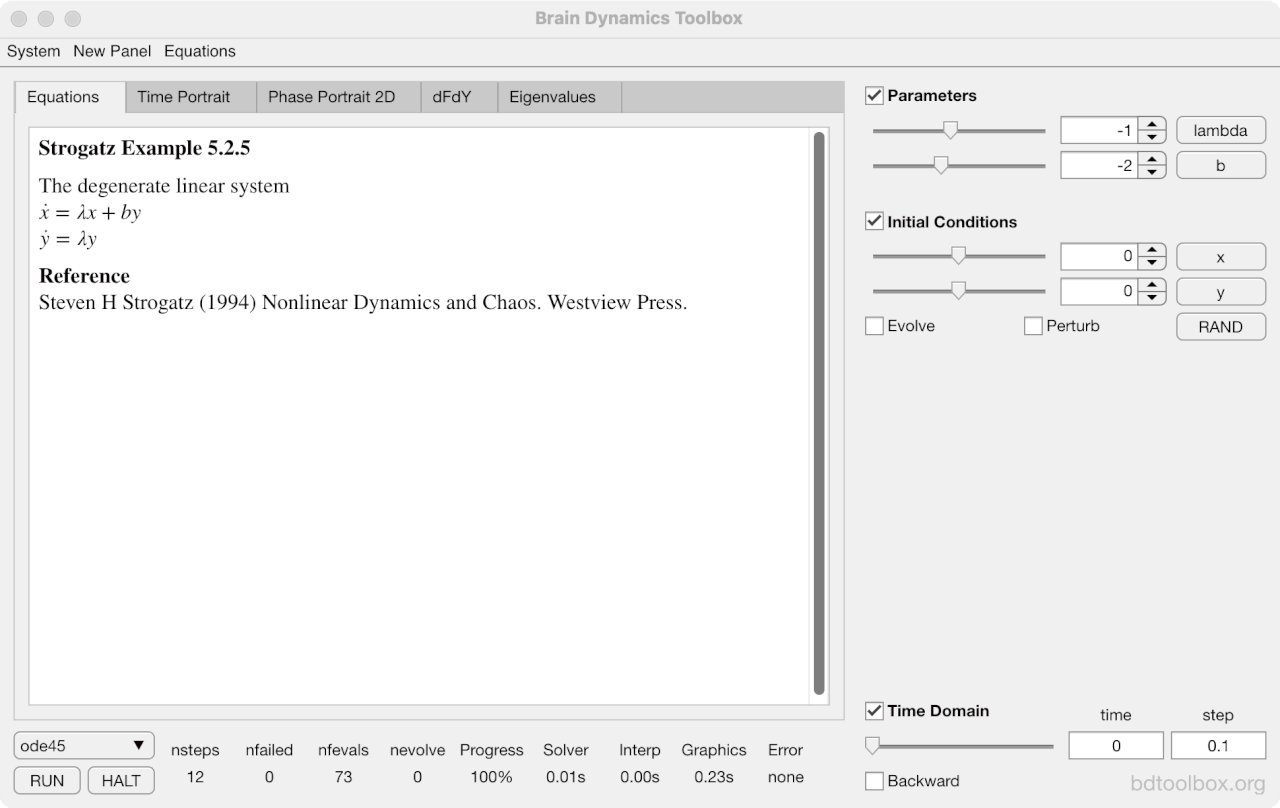Example 5.2.5. A degenerate node has only one eigenvector.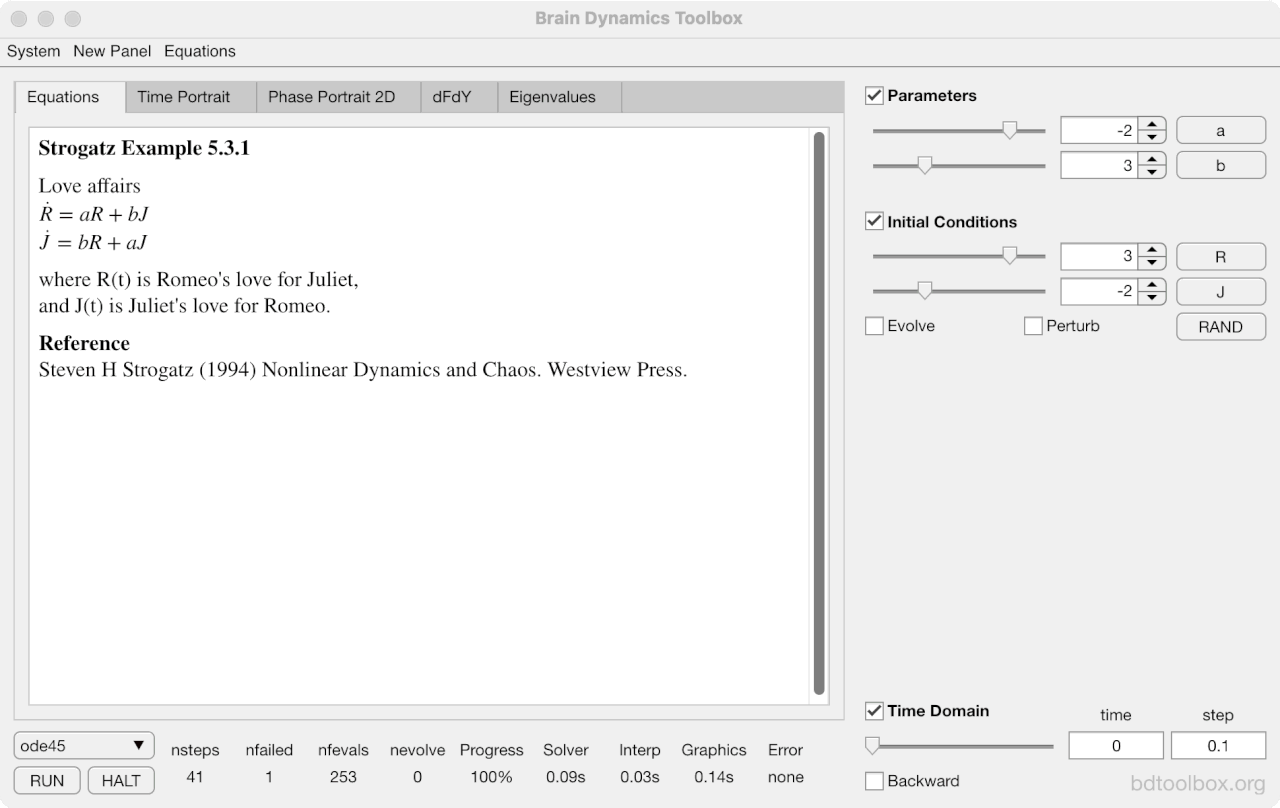Example 5.3.1. Love affairs.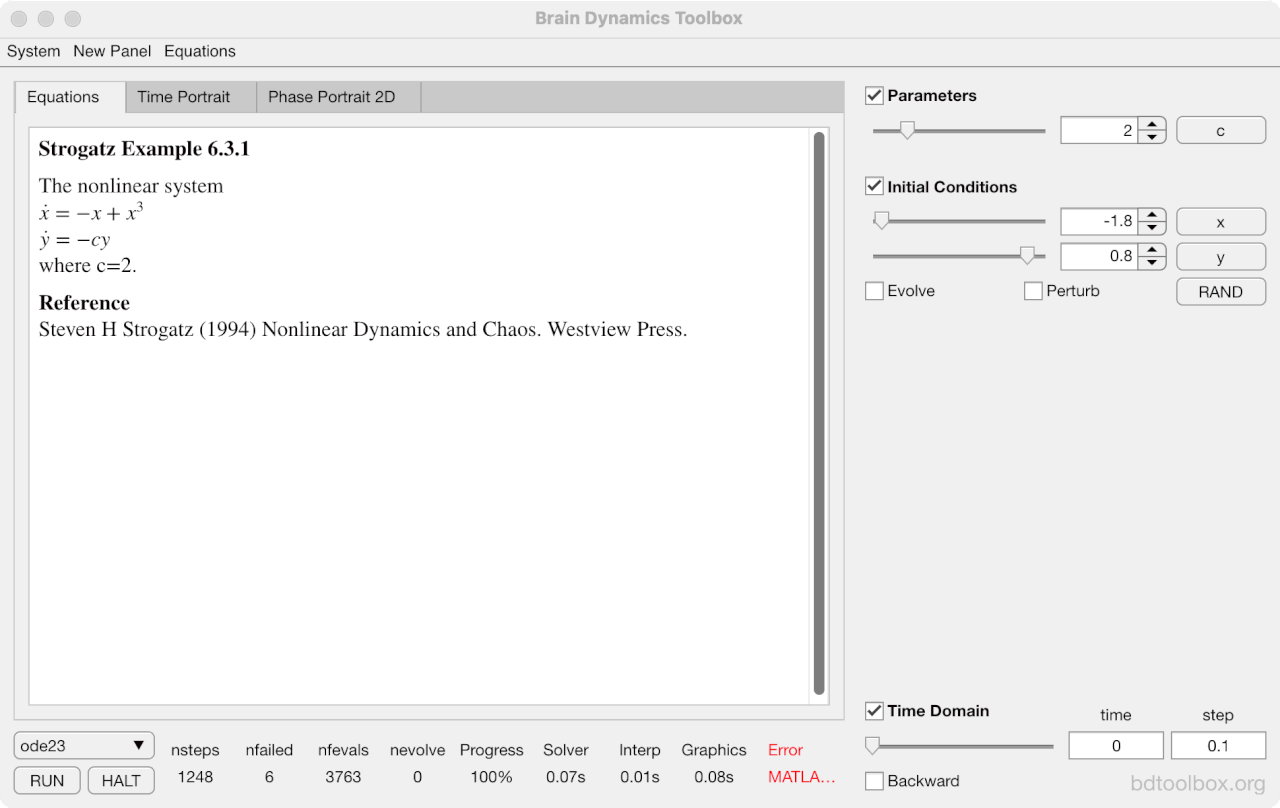Example 6.3.1. Saddles and stable nodes.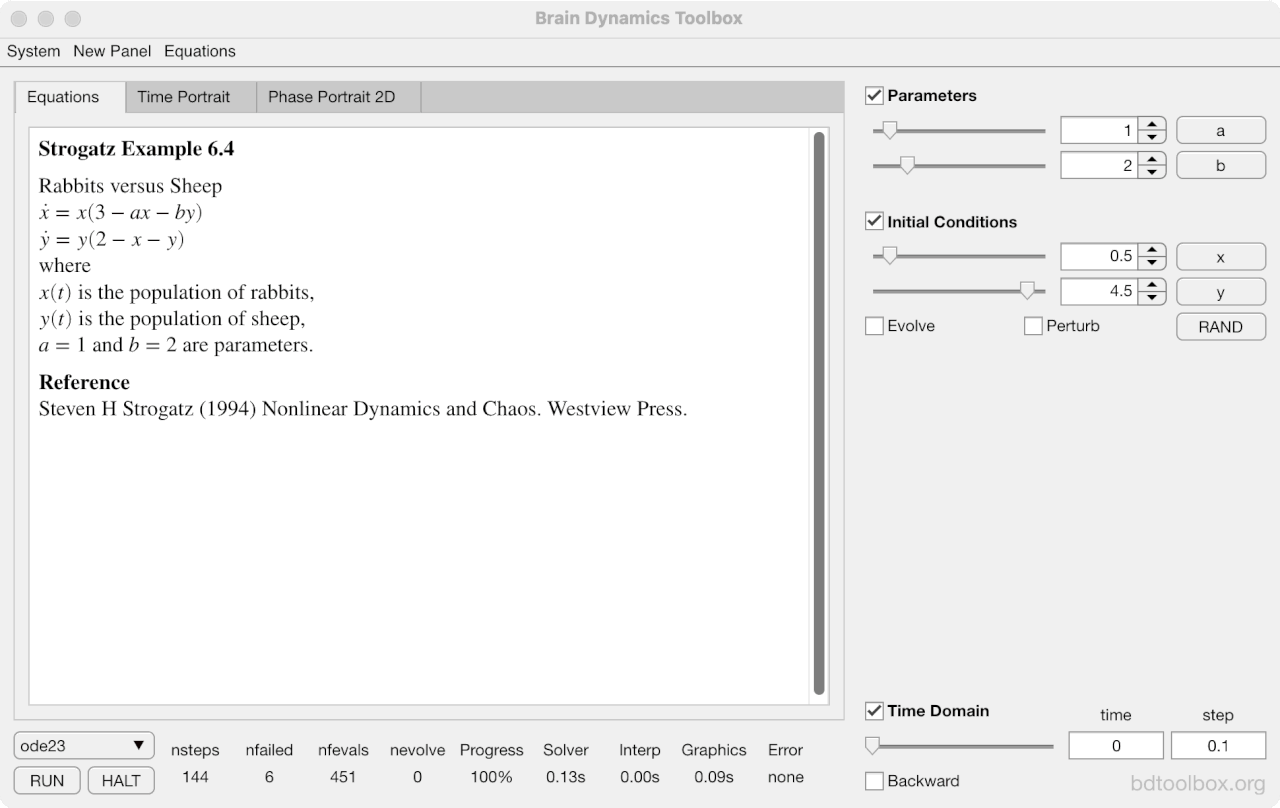Example 6.4. Rabbits versus Sheep.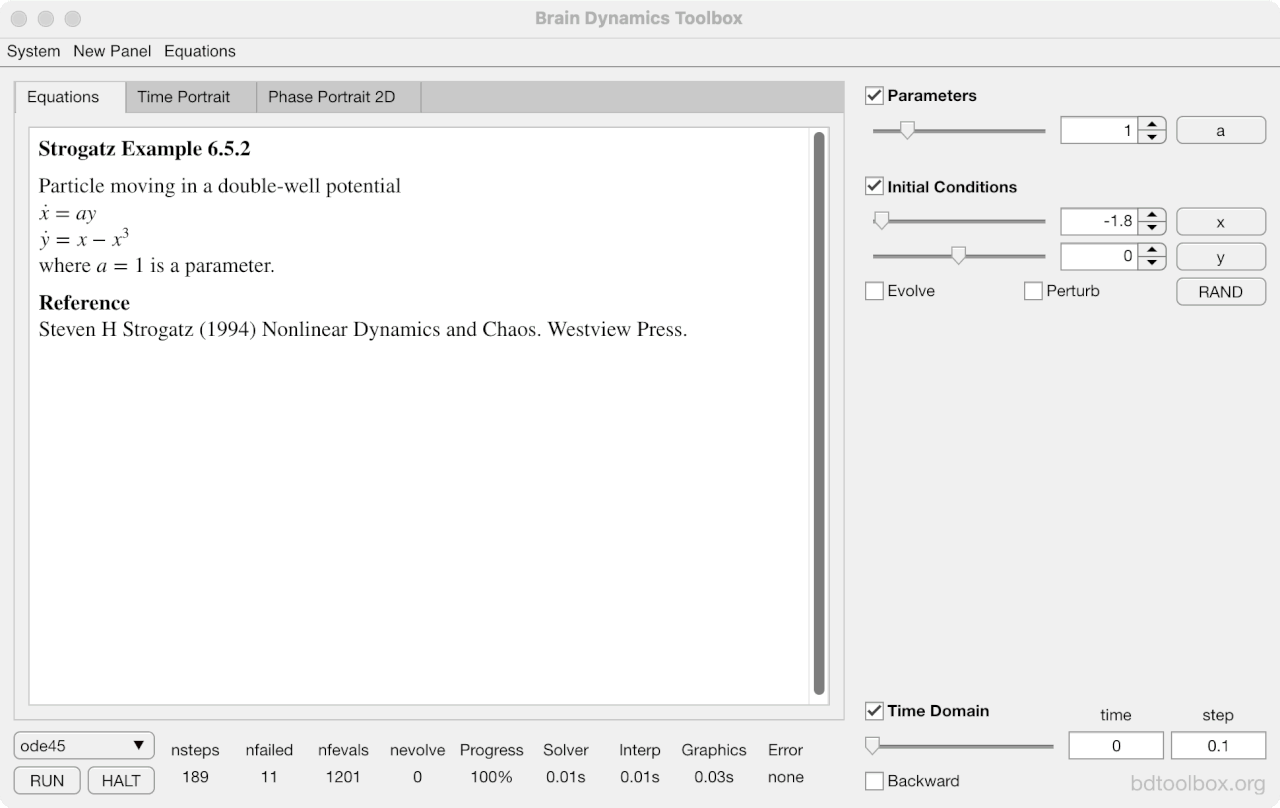Example 6.5.2. Particle moving in a double well potential.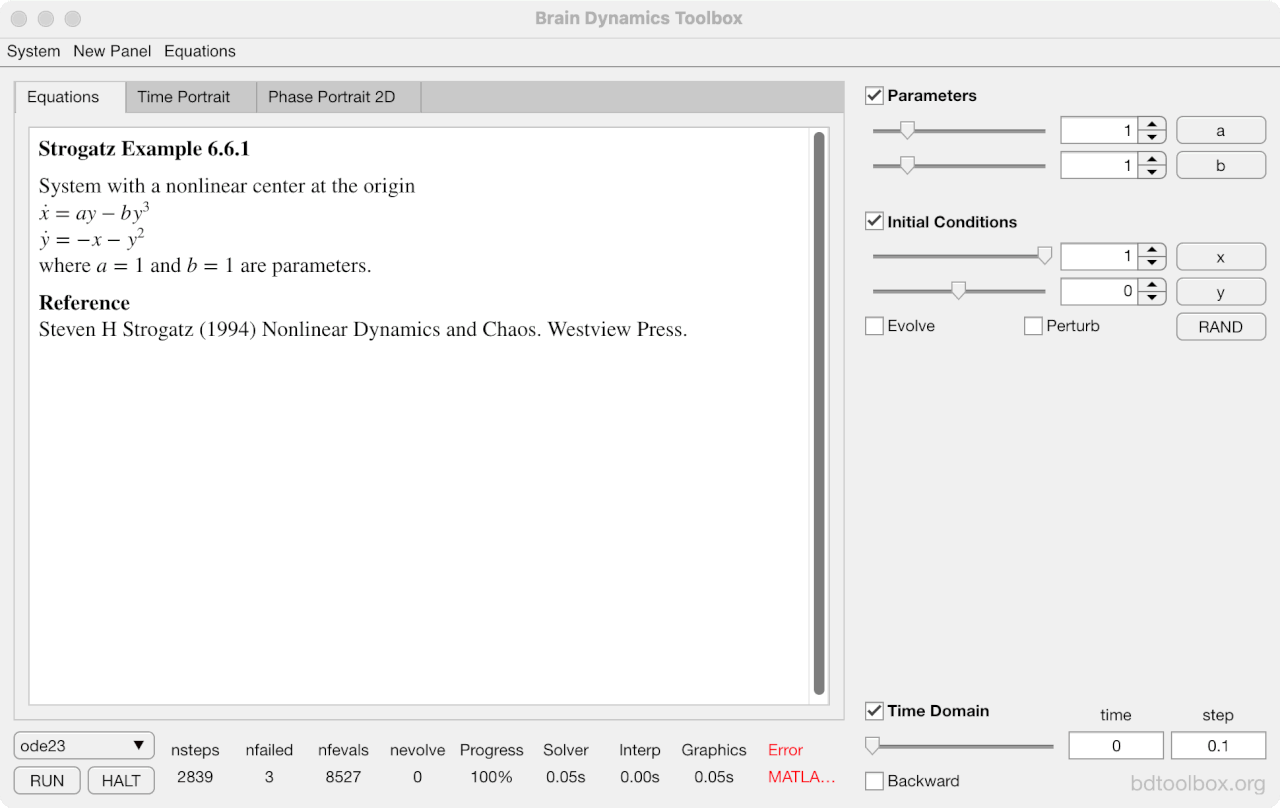Example 6.6.1. System with a nonlinear centre at the origin.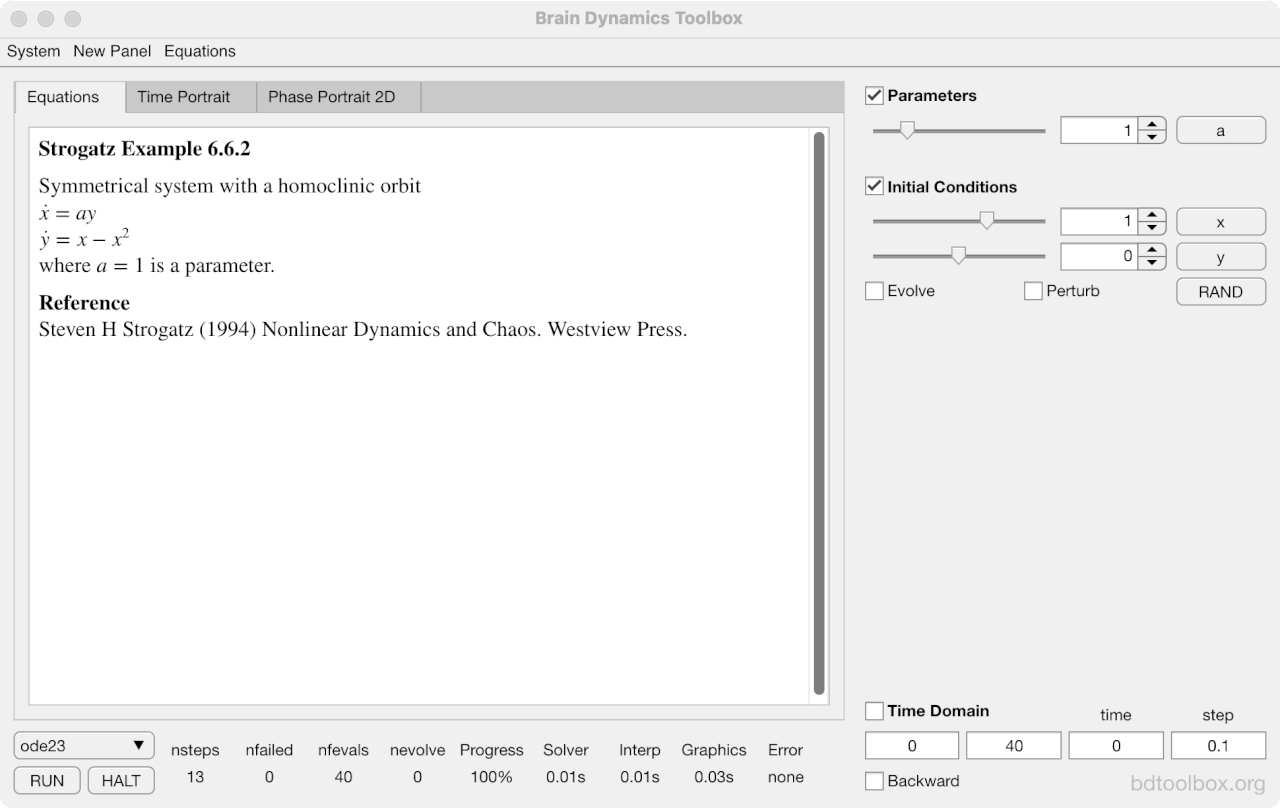Example 6.6.2. Symmetrical system with a homoclinic orbit.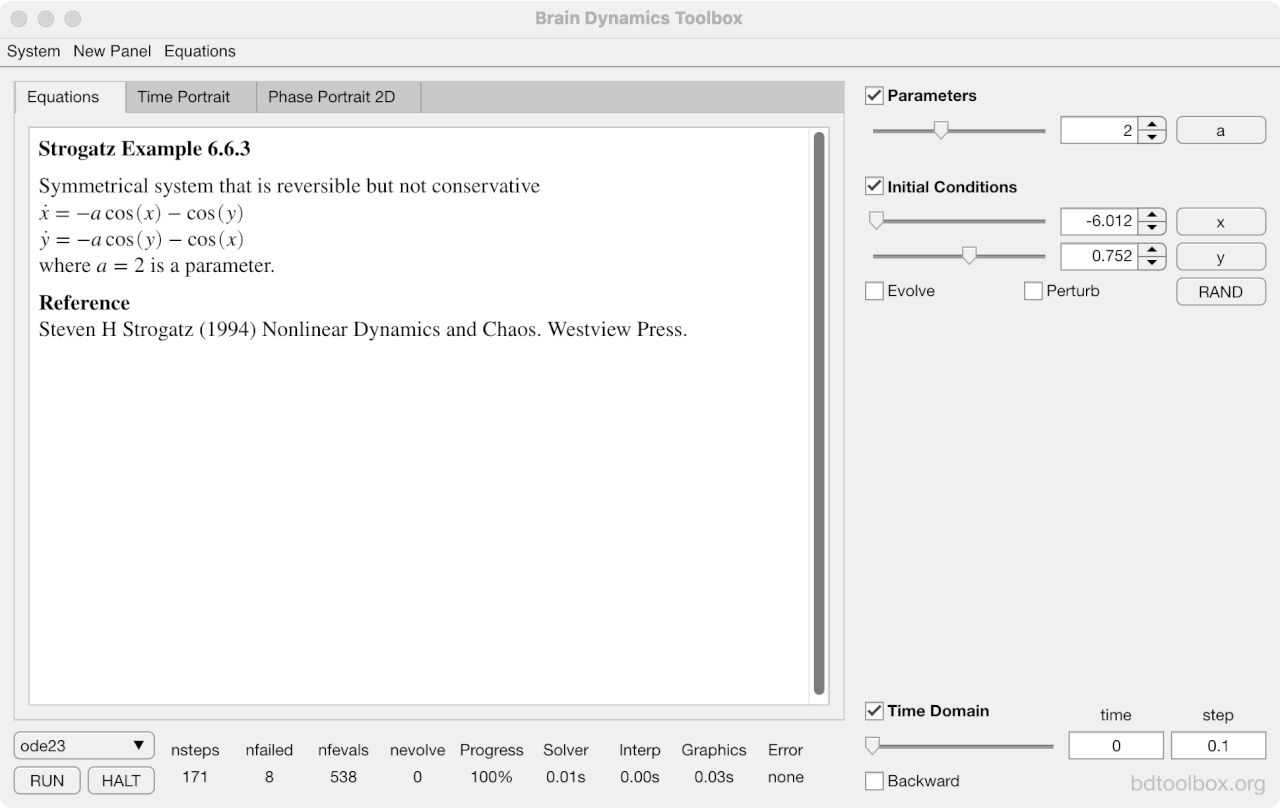Example 6.6.3. A symmetrical system that is reversible but not conservative.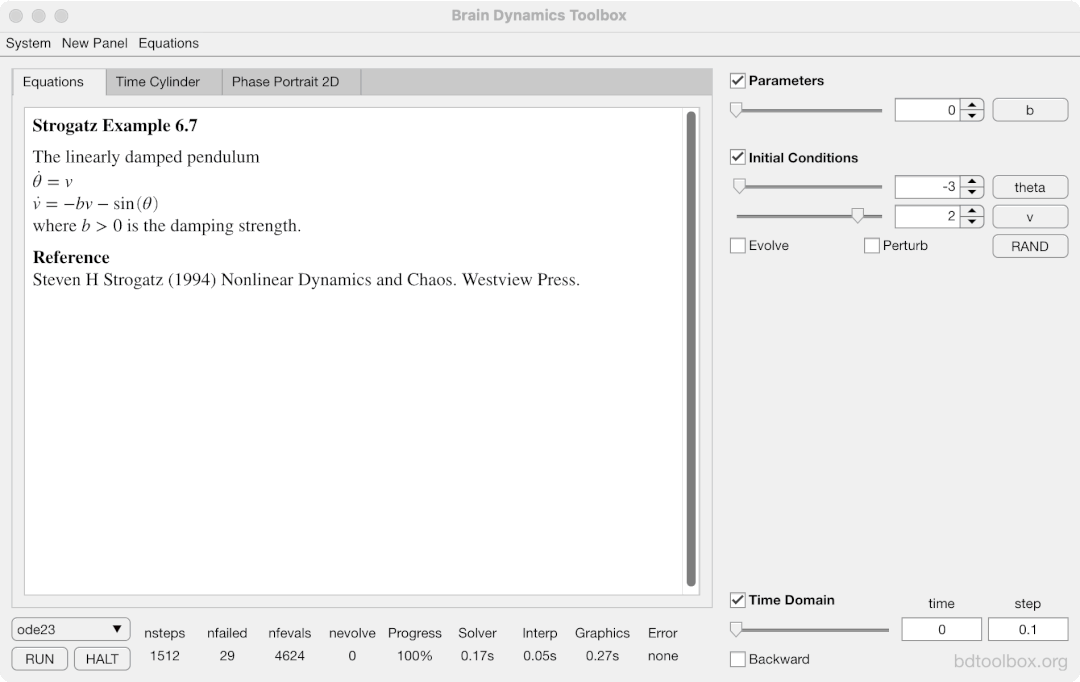Example 6.7. The linearly damped pendulum.